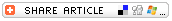Home >> A Tsl >> Archives >> 06 1 >> 'Doubles Plus One' Math Game

#"Doubles Plus One" Math GameSubjects

• Arithmetic

• K-2
• 3-5

Brief Description

Students practice doubling numbers with this fun, fast-paced dice game.

Objectives

Students practice doubling numbers with this fun, fast-paced dice game.

Keywords

double, dice, game

• one die numbered 1 to 6 per pair of students or one die "doctored" numbered 4 to 9 per pair
• two different colored crayons
• one game board per pair of students (see instructions for creating the game board in the Before the Lesson section below)

The Lesson

Before the Game
The gameboard for this game is simple to make. Fold a paper to create a 12-square gameboard; or draw a 12-square board on a sheet of paper.

In this game, students will be rolling a die, then doubling (or doubling and then adding 1) the number on that die.

• If you are using a die with the numbers 1 to 6, number the squares on the gameboard from 2 to 13, one number per square; be sure all twelve numbers appear on the gameboard.
• If you are using a die that has been doctored to have the numbers 4 to 9, number the squares on the gameboard from 8 to 19; be sure all twelve numbers appear on the gameboard.

Playing the Game
Introduce, then practice with your students, the concept of doubling. Then introduce and practice the concept of doubling then adding 1 to the answer.

• The number 4 doubled becomes 8.
• If you double 4, then add 1, the answer is 9.

Arrange students into pairs. Each pair can make their own 12-square gameboard (see instructions in the Before the Game section above). Provide a die for each pair of students.

• If you teach young students in grades 3 and under, you might use a regular game die, which is numbered from 1 to 6.
• If you teach students in grades 3 and above, you might "doctor" the die so that it has the numbers 4 to 9 on it.

The first player rolls the die and doubles the number that appears on it -- or that player can doubles plus one the number on the die.

For example, if a 3 is rolled on the die, the student has the choice of a doubling the 3 (for an answer of 6 or doubling plus 1 for an answer of 7. The player who rolled the 3 can choose to use her/his crayon to draw an X on the gameboard on either the number 6 or 7.

Next, the second player does the same thing.

As the game goes on, students will begin rolling a number for which the doubled number and the doubles-plus-one number are already X'd. That player is unable to X a number, so play turns over to the opponent.

The game ends when all twelve numbers on the gameboard are X'd. Each player counts up the number of X's made in his/her color. The winner is the player who has X'd the most numbered squares.

Assessment

As students play the game, circulate to see how fluent they are in understanding the concept and the rules. You can create a written work sheet to further assess students' grasp of the skills of doubling and doubling then adding one.

Submitted By

Patti Woodhull, Vista Fundamental School in Simi Valley, California

Education WorldÂ®